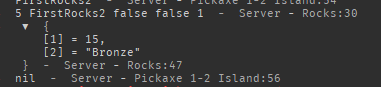# Problem with module script

Hello, I am having trouble with module script.

my issue is that I have a table that I need to access from the server script. the table is okay when I print it from the module script, but when I print it on the server script it is nil for some reason

This is module script

``````local Rocks = {
["FirstRocks"] = {
["Damaged"] = {5},
["Broke"] = {},
["Ores"] = {
 = {3,"Silver",3,5}, -- Chance,What,Weight (min-max)
 = {97,"Bronze",1,3}
}
},
["FirstRocks2"] = {
["Damaged"] = {10},
["Broke"] = {},
["Ores"] = {
 = {3,"Silver",3,5}, -- Chance,What,Weight (min-max)
 = {97,"Bronze",1,3}
}
},
["FirstRocks3"] = {
["Damaged"] = {15},
["Broke"] = {},
["Ores"] = {
 = {5,"Silver",3,5}, -- Chance,What,Weight (min-max)
 = {95,"Bronze",1,3}
}
}
}

function Rocks.Damaged(Damage,WhichRock,Broken,GamePass,Rebirths)
print(Damage,WhichRock,Broken,GamePass,Rebirths)
local random = Random.new():NextNumber(0, 100)
local counter = 0
local Ore = ""
for i,v in pairs(Rocks[WhichRock.Name]["Ores"]) do
counter += v
if random <= counter then
Ore = v
end
end
local rocks = (Rocks[WhichRock.Name]["Damaged"] + Damage) * Rebirths

local STATS = {
rocks,
Ore
}

print(STATS)
return STATS
end)
end

return Rocks
``````

this is server script:

and this is the output1 Like

Hi, can we see the server script cause there might be something wrong there you will have to provide the full code of the server script for us to know the error.alright!

``````task.wait(0.3)
local RS = game:GetService("ReplicatedStorage")
local SS = game:GetService("ServerStorage")

local RockRespawn = require(game:GetService("ServerScriptService"):WaitForChild("RockRespawn"))
local Pickaxe = RS:WaitForChild("RemoteEvents"):WaitForChild("First6Pickaxe")
local Module = require(game:GetService("ReplicatedStorage"):WaitForChild("Rocks"))

Pickaxe.OnServerEvent:Connect(function(player,target)
if target.Name ~= "RockHitbox" then Pickaxe:FireClient(player) return end
local char = player.Character
if not char then return end
local Handle
if player:WaitForChild("TempValues"):WaitForChild("PickaxeEquipped").Value == false then return end
for i,v in pairs(char:GetChildren()) do
if not char then return end
if v:IsA("Tool") then
if v:FindFirstChild("Handle") then
Handle = v.Handle
end
end
end
if not Handle then return end
if (Handle.Position - target.Position).Magnitude > 10 then	Pickaxe:FireClient(player) return end
if (Handle.Position - target.Position).Magnitude <= 10 then
local Damage local CriticalChance local CriticalBoost local Speed local Rebirths = player:WaitForChild("leaderstats"):WaitForChild("Rebirths").Value local WhichRock
Damage = tonumber(PickaxeStats) CriticalChance = tonumber(PickaxeStats) CriticalBoost = tonumber(PickaxeStats) Speed = tonumber(PickaxeStats)
local critical = false
local random = math.random(0,100)
if CriticalBoost >= random then
critical = true
end
local valuesFolder local TimeToWait
if player then valuesFolder = player.ValuesFolder end

local PetFolder = workspace.PlayerPets:FindFirstChild(player.Name)
if not PetFolder then return end
for i,v in pairs(PetFolder:GetChildren()) do
if v:IsA("Model") then
Damage += v.Folder.Damage.Value
end
end
WhichRock = target.Parent
local Broken = false local GamePass = false
WhichRock.HP.Value -= Damage
if Damage >= WhichRock.HP.Value then
Broken = true
end
if player:WaitForChild("leaderstats"):WaitForChild("Rebirths").Value == 0 then Rebirths += 1 end
print(WhichRock)
local newTable = Module.Damaged(Damage,WhichRock,Broken,GamePass,Rebirths)
print(newTable)
if newTable ~= nil or newTable ~= '' then
print("WOWWWW YOU GOT"..newTable)
end
if Broken then
target.Parent.HP.Value = target.Parent.MaxHP.Value
RockRespawn.Reposition(target.Parent.Which.Value,target)
end
Pickaxe:FireClient(player)
end
end)
end)
``````

if you look at this code you can see the part where you printed and where you returned, the thing is that you are returning in the task.spawn function, not the Rocks.Damaged Function

1 Like

I am so dumb:) Thanks! (char limit)

1 Like